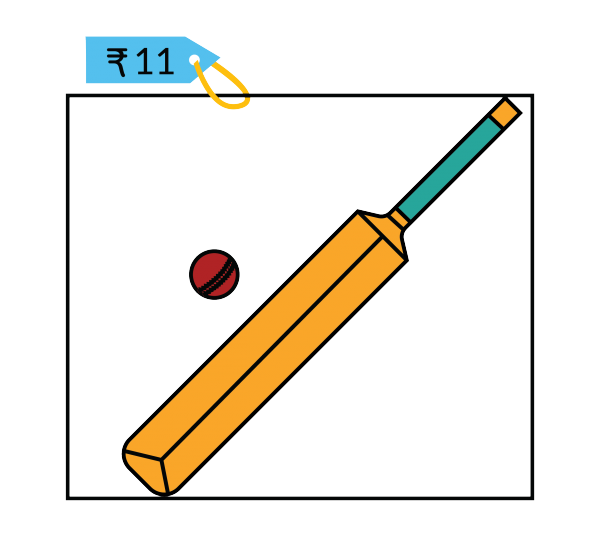Math Puzzles

# PUZZLE SOLUTION P2

1k views

The cost of the bat is not ₹10 although it is mentioned that the bat costs ₹10 more than the ball.

Let’s revisit the question:

A toy bat and a toy ball together cost ₹11. The cost of the bat is ₹10 more than the ball. What is the cost of the bat?It is quite easy to assume that the bat is ₹10 and the ball is ₹1 which would make the combo equal to ₹11. But, the given condition in the question will not be met if we assume these values.

The bat costs ₹10 more than the ball.

Since the ball is ₹1, then the cost of the bat alone would become ₹(1+10)=11 which is not correct because, the price of both together should be ₹11.

Here’s how to analyse this situation.

Let us assume that the ball costs “R” rupees. Then the bat would cost ₹(R+10)

So, if we look at the total cost,

R+(R+10) = 11 => 2R= 11 – 10

2R=1 => R= ½ = 0.5

Since R represents the cost of the ball which is half a rupee (50p), the cost of the bat would be ₹10.50

Congratulations if you got the correct answer!

Related Articles
Award-winning math curriculum, FREE for a year